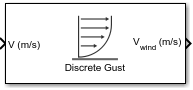# Discrete Wind Gust Model

Generate discrete wind gust

## Library

Environment/Wind

•## Description

The Discrete Wind Gust Model block implements a wind gust of the standard “1-cosine” shape. This block implements the mathematical representation in the Military Specification MIL-F-8785C . The gust is applied to each axis individually, or to all three axes at once. You specify the gust amplitude (the increase in wind speed generated by the gust), the gust length (length, in meters, over which the gust builds up) and the gust start time.

The Discrete Wind Gust Model block can represent the wind speed in units of feet per second, meters per second, or knots.

The following figure shows the shape of the gust with a start time of zero. The parameters that govern the gust shape are indicated on the diagram.The discrete gust can be used singly or in multiples to assess airplane response to large wind disturbances.

The mathematical representation of the discrete gust is

`${V}_{wind}=\left\{\begin{array}{lll}0\hfill & x<0\hfill & \hfill \\ \frac{{V}_{m}}{2}\left(1-\mathrm{cos}\left(\frac{\pi x}{{d}_{m}}\right)\right)\hfill & 0\le x\le {d}_{m}\hfill & \hfill \\ {V}_{m}\hfill & x>{d}_{m}\hfill & \hfill \end{array}$`

where Vm is the gust amplitude, dm is the gust length, x is the distance traveled, and Vwind is the resultant wind velocity in the body axis frame.

## Parameters

Units

Define the units of wind gust.

Units

Wind

Altitude

`Metric (MKS)`

Meters/second

Meters

`English (Velocity in ft/s)`

Feet/second

Feet

`English (Velocity in kts)`

Knots

Feet

Gust in u-axis

Select to apply the wind gust to the u-axis in the body frame.

Gust in v-axis

Select to apply the wind gust to the v-axis in the body frame.

Gust in w-axis

Select to apply the wind gust to the w-axis in the body frame.

Gust start time (sec)

The model time, in seconds, at which the gust begins.

Gust length [dx dy dz] (m or f)

The length, in meters or feet (depending on the choice of units), over which the gust builds up in each axis. These values must be positive.

Gust amplitude [ug vg wg] (m/s, f/s, or knots)

The magnitude of the increase in wind speed caused by the gust in each axis. These values may be positive or negative.

## Inputs and Outputs

InputDimension TypeDescription

First

Contains the airspeed in units selected.
OutputDimension TypeDescription

First

Contains the wind speed in units selected.

## Examples

See ```Discrete Wind Gust Model``` in `aeroblk_HL20` for an example of this block.

## Reference

U.S. Military Specification MIL-F-8785C, 5 November 1980.

## Extended Capabilities

### C/C++ Code GenerationGenerate C and C++ code using Simulink® Coder™.

#### Introduced before R2006a

##### SupportGet trial now